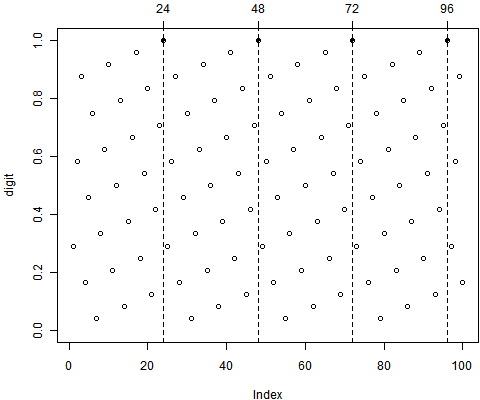> digit = ((1:100) * 0.29166666)%%1 # 整除1之后的“余数”
> plot(digit, ylim = c(0, 1))
> idx = which((1 - digit) < 1e-05 | (digit - 0) < 1e-05) # 与0或1很靠近时
> points(idx, digit[idx], pch = 20)
> abline(v = idx, lty = 2)
> axis(3, idx)
> idx * 0.29166666
  7 14 21 28